Get instant live expert help with Excel or Google Sheets“My Excelchat expert helped me in less than 20 minutes, saving me what would have been 5 hours of work!”

#### Post your problem and you’ll get Expert help in seconds.

Your message must be at least 40 characters
Our professional Expert are available now. Your privacy is guaranteed.

# Create and Use a Dynamic Worksheet Reference in Excel

We can create a dynamic worksheet so that cell references can be variable instead of hand-keying them. A dynamic worksheet can be created with the INDIRECT function. The easy steps below will walk through the process.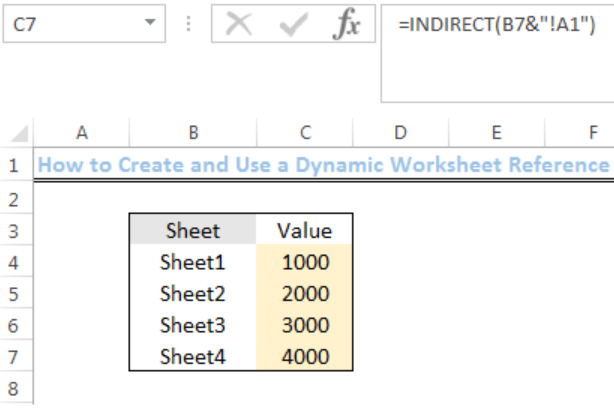Figure 1: Result of the Dynamic Worksheet Reference

## Syntax

`=INDIRECT(sheet_name&"!A1”)`

## Formula

`=INDIRECT(B4&"!A1")`

## Setting up the Data

We will set up the data by doing the following:

• We will name the first sheet as reference worksheet by right-clicking the plus sign enclosed in a circle as shown in figure 2 and click on rename
• We will create Sheet1 to Sheet4 by clicking on the plus sign enclosed in a circle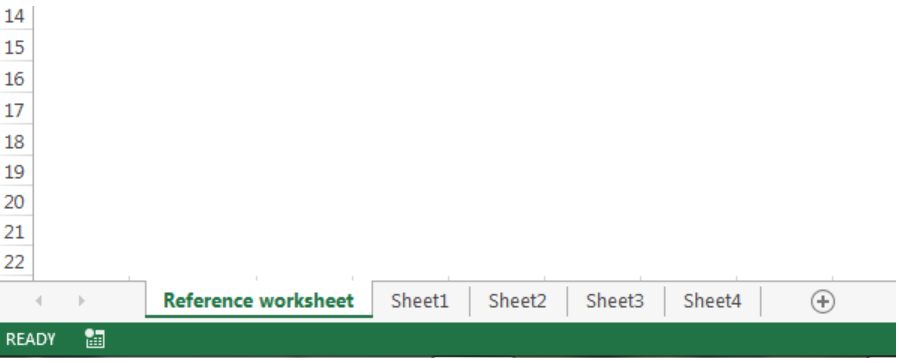Figure 2: Dynamic reference worksheet

• We will input the following values into Cell A1 in Sheet1, Sheet2, Sheet3, and Sheet4 respectively: 1000, 2000, 3000, and 4000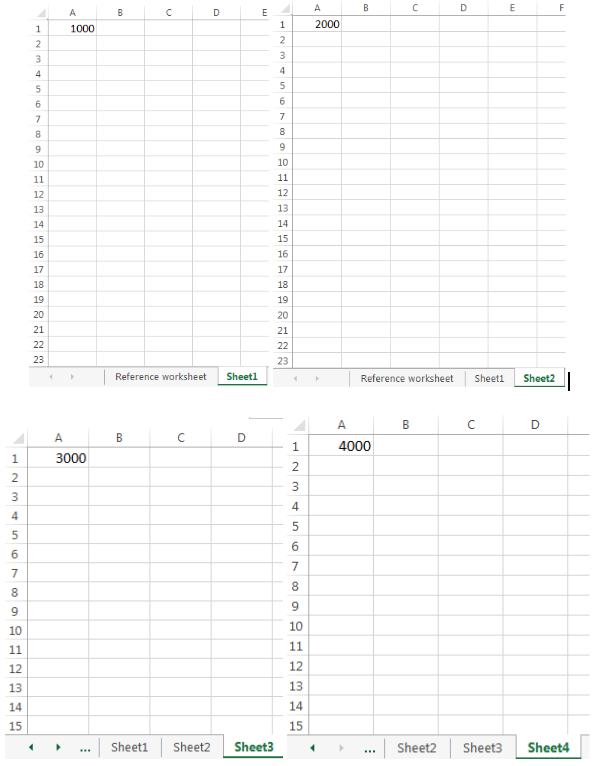Figure 3: Sheet 1 to Sheet4 containing Values in Cell A1

• We will input the data as shown in figure 4 into the reference worksheet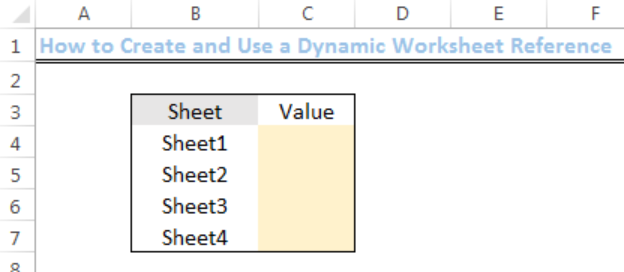Figure 4: Inputting the sheet names into the reference worksheet

## Using a Dynamic Worksheet Reference

• We will input the formula below into Cell C4 of the REFERENCE WORKSHEET: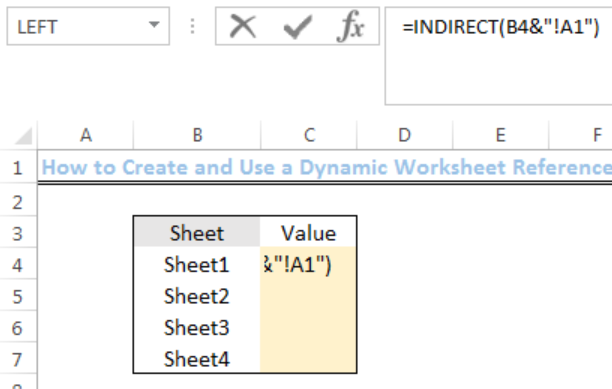Figure 5: How to Use a Dynamic Worksheet Reference

• We will press enter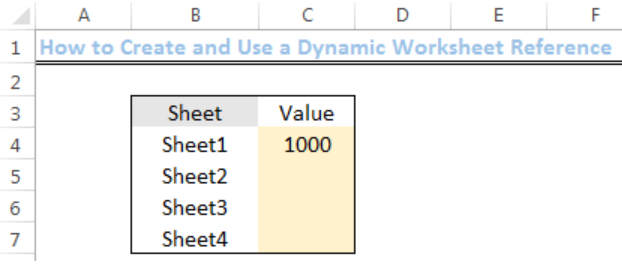Figure 6: How to Use a Dynamic Worksheet Reference

• We will use the drop-down feature to get the values for the other sheets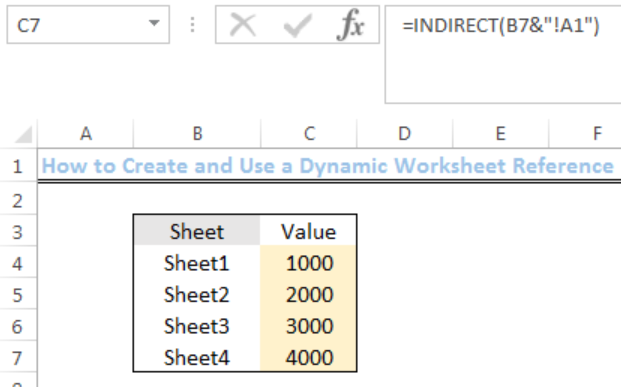Figure 7: Result of the Dynamic Worksheet Reference

## Explanation

The INDIRECT function works by evaluating text as a worksheet reference. Here, the name of each sheet is joined to the cell reference (A1) using concatenation:

`=INDIRECT(B4&"!A1")`

Once concatenation is done, the result is:

`=INDIRECT("Sheet1!A1")`

The INDIRECT function will recognize the value in Cell A1 of Sheet1 and return the value. The same applies when we use the drop-down feature for the other sheets.

## Instant Connection to an Expert through our Excelchat Service

Most of the time, the problem you will need to solve will be more complex than a simple application of a formula or function. If you want to save hours of research and frustration, try our live Excelchat service! Our Excel Experts are available 24/7 to answer any Excel question you may have. We guarantee a connection within 30 seconds and a customized solution within 20 minutes.

### Did this post not answer your question? Get a solution from connecting with the expert.Another blog reader asked this question today on Excelchat:
Solution examplesI have a google sheet that takes information from a google form, that creates a row every time a form is submitted. Since it is a new row that is created, rather than filling in the next row down, the formulas I have applied to some of the columns do not work. The same is true when I try and link the data to another sheet to bypass this problem. This is probably quite a general functionality issue so I won't send the actual document but let me know if you need it
Solved by F. F. in 13 minsHello, I need help regarding indirect formula of excel. 1. What is the purpose of using this formula? 2. If we use this formula with sum formula, then why we are using this with sum formula, we can also perform addition from different sheets without indirect formula ?
Solved by O. D. in 21 minsI want to dynamic access a sheet/cell from a closed file using INDEX. But when I try to "&A3&" for sheet name I forces me to select a file and then populates the sheet name instead of making it dynamically INDEX('/Users/me/Desktop/["&A2&"]"&A3&"'!A:A,1,1)
Solved by G. H. in 20 minsI need explanation for the excel formula below... =IF(ISERROR(SUMIFS(INDIRECT("'"&BE\$6&"'!"&"\$G:\$G"),INDIRECT("'"&BE\$6&"'!"&"\$R:\$R"),\$A39,INDIRECT("'"&BE\$6&"'!"&"\$F:\$F"),"<>52711",INDIRECT("'"&BE\$6&"'!"&"\$C:\$C"),10)),0,SUMIFS(INDIRECT("'"&BE\$6&"'!"&"\$G:\$G"),INDIRECT("'"&BE\$6&"'!"&"\$R:\$R"),\$A39,INDIRECT("'"&BE\$6&"'!"&"\$F:\$F"),"<>52711",INDIRECT("'"&BE\$6&"'!"&"\$C:\$C"),10))+IF(ISERROR(SUMIFS(INDIRECT("'"&BE\$4&"'!"&"\$A\$30:\$EO\$30"),INDIRECT("'"&BE\$4&"'!"&"\$A\$4:\$EO\$4"),BE\$5,INDIRECT("'"&BE\$4&"'!"&"\$A\$5:\$EO\$5"),\$A39)),0,SUMIFS(INDIRECT("'"&BE\$4&"'!"&"\$A\$30:\$EO\$30"),INDIRECT("'"&BE\$4&"'!"&"\$A\$4:\$EO\$4"),BE\$5,INDIRECT("'"&BE\$4&"'!"&"\$A\$5:\$EO\$5"),\$A39))+IF(ISERROR(SUMIF(INDIRECT("'"&BE\$6&" "&\$A\$31&"'!"&"H:H"),\$A39,(INDIRECT("'"&BE\$6&" "&\$A\$31&"'!"&"I:I")))),0,SUMIF(INDIRECT("'"&BE\$6&" "&\$A\$31&"'!"&"H:H"),\$A39,(INDIRECT("'"&BE\$6&" "&\$A\$31&"'!"&"I:I"))))
Solved by V. A. in 20 mins## Subscribe to Excelchat.coAnother blog reader asked this question today on Excelchat: